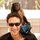# Chart Type - Histogram

Histograms are useful for showing the distribution of a data set while aggregating up to see more general trends. Here is the SQL code (in a handy parameterized snippet!) for binning numeric data and creating histograms from columns.

Snippet Name: histogram(table_name,column,bin_size)

```select
[column] - mod([column], [bin_size]) as value
, [column] - mod([column], [bin_size]) || ' - ' || [column] + ([bin_size] - mod([column], [bin_size])) as label
, count(1)
from
[table_name]
group by
1
, 2
order by
1 asc
```

Alternatively, you can create custom bin sizes that vary in width using a case when statement

```select
case
when [column] < 100
then '100'
when [column] < 500
then '500'
when [column] < 1000
then '1000'
else '> 1000'
end as [column]
, count(1)
from
[table_name]
group by
1
```